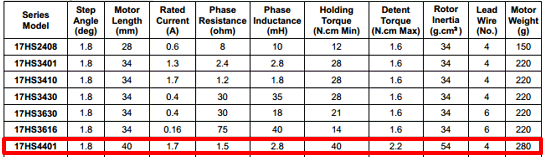# What is the correct method to set the current limit?

My motor (17HS4401) have 1.7A as rated currentand i want to know the correct method to set Vref :
1-Method
1.7A / 2 = 0.85v (vref)

2-Method
The motor use the full step mode so the current will be 0.7 times (70%) the current limit :
current limit = 1.7A / 0.7 = 2.43A
Vref = current limite / 2 ==> 2.43/2 2.43 = 1.21V

3-Method
vref = Inom * 5 * Rsense *0.7 so Vref = 1.7 * 5 * 0.1 * 0.7 = 0.6V

what do you think?

You did not specify what stepper motor driver you are using; however, from your previous posts and the equations you used here, it sounds like you are using a DRV8825 carrier.

If that is the case, your first two equations look correct (depending on if you are accounting for the 70% in full-step mode); however, as Derrill mentioned in your previous thread, the DRV8825 carrier should be limited to 1.5A per phase without additional cooling. A VREF setting of 0.75V would set the current limit to 1.5A per phase. If you are only using full-step mode, you can increase the VREF to about 1.05V. This results in a current limit of 2.1A per phase, but since both coils are always on and limited to approximately 70% of the current limit setting in full-step mode, it only ever reaches about 1.5A per phase.

By the way, to correct your 3rd equation, you would need to move the 0.7 to the VREF side of the equation (VREF × 0.7 = I × 5 × Rsense). Then it simplifies down to your second equation.

Yes, I use DRV8825 with additional cooling.
Please can you tell how to calculate vref in my case: bipolar stepper motor (17HS4401) 1.7A as rated current like mentioned in the datasheetThe equation stays the same; if you are adding cooling, you will need to figure out what current limit to set based on your setup and what it can handle.

Brandon

But wich method i will use , my motor is bipolar and have 1.7A as rated current

The difference between what you labeled as method 1 and method 2 is just whether or not you account for the driver only using 70% of the current limit when in full step mode. I recommend starting with the lower current limit (i.e. the value calculated in your 1st method) and testing to see if that will work for you. If you need more torque for your system and are only using full step mode, raising it to the value you calculate using your 2nd method might help.

Brandon

For the same motor but with a A4988 driver would the formulas be similar?

Thanks

Hello, Sanyamo.

You can find the VREF formula for current limiting for our A4988 carriers in the “Current limiting” section of the driver’s product page.

Please note, other manufacturer’s drivers might have different formulas based on the components they use on their carriers.

-Derrill